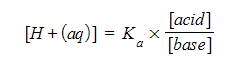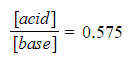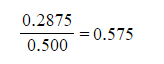# Edexcel A Level Chemistry:复习笔记5.2.7 Buffers

### Action of a Buffer Solution

• A buffer solution is a solution which resists changes in pH when small amounts of acids or alkalis are added
• A buffer solution is used to keep the pH almost constant
• A buffer can consists of weak acid - conjugate base or weak base - conjugate acid

#### Ethanoic acid & sodium ethanoate as a buffer

• A common buffer solution is an aqueous mixture of ethanoic acid and sodium ethanoate
• Ethanoic acid is a weak acid and partially ionises in solution to form a relatively low concentration of ethanoate ions• Sodium ethanoate is a salt which fully ionises in solution• There are reserve supplies of the acid (CH3COOH) and its conjugate base (CH3COO-)
• The buffer solution contains relatively high concentrations of CH3COOH (due to partial ionisation of ethanoic acid) and CH3COO- (due to full ionisation of sodium ethanoate)
• In the buffer solution, the ethanoic acid is in equilibrium with hydrogen and ethanoate ions• When H+ ions are added:
• The equilibrium position shifts to the left as H+ ions react with CH3COO- ions to form more CH3COOH until equilibrium is re-established
• As there is a large reserve supply of CH3COO- the concentration of CH3COO- in solution doesn’t change much as it reacts with the added H+ ions
• As there is a large reserve supply of CH3COOH the concentration of CH3COOH in solution doesn’t change much as CH3COOH is formed from the reaction of CH3COO- with H+
• As a result, the pH remains reasonably constantWhen hydrogen ions are added to the solution the pH of the solution would decrease; However, the ethanoate ions in the buffer solution react with the hydrogen ions to prevent this and keep the pH constant

• When OH- ions are added:
• The OH- reacts with H+ to form water

OH- (aq) + H+  (aq) → H2O (l)

• The H+ concentration decreases
• The equilibrium position shifts to the right and more CH3COOH molecules ionise to form more H+and CH3COO- until equilibrium is re-established

CH3COOH (aq) → H+ (aq) + CH3COO- (aq)

• As there is a large reserve supply of CH3COOH the concentration of CH3COOH in solution doesn’t change much when CH3COOH dissociates to form more H+ ions
• As there is a large reserve supply of CH3COO- the concentration of CH3COO- in solution doesn’t change much
• As a result, the pH remains reasonably constantWhen hydroxide ions are added to the solution, the hydrogen ions react with them to form water; The decrease in hydrogen ions would mean that the pH would increase however the equilibrium moves to the right to replace the removed hydrogen ions and keep the pH constant

### Buffers & pH Calculations

• The pH of a buffer solution can be calculated using:
• The Ka of the weak acid
• The equilibrium concentration of the weak acid and its conjugate base (salt)

• To determine the pH, the concentration of hydrogen ions is needed which can be found using the equilibrium expression• To simplify the calculations, logarithms are used such that the expression becomes:• Since -log10 [H+] = pH, the expression can also be rewritten as:• This is known as the Hendersen-Hasselbalch equation

#### Worked Example

Calculate the pH of a buffer solution containing 0.305 mol dm-3 of ethanoic acid and 0.520 mol dm-3 sodium ethanoate.

The Ka of ethanoic acid  = 1.74 × 10-5 mol dm-3 at 298 K

Ethanoic acid is a weak acid that ionises as follows:

CH3COOH (aq) ⇌ H+ (aq) + CH3COO- (aq)

Step 1: Write down the equilibrium expression to find KaStep 2: Rearrange the equation to find [H+]Step 3: Substitute the values into the expression= 1.02 x 10-5 mol dm-3

Step 4: Calculate the pH

pH = - log [H+]

= -log 1.02 x 10-5

= 4.99

#### How to make a buffer solution with a required pH

• To make a buffer solution with a pH of less than 7, you need to use a mixture of a weak acid and its conjugate base
• Conversely, you can make a buffer solution with a pH greater than 7 by using a mixture of a weak base and its conjugate acid
• Imagine we want to make a buffer solution with a pH of 5.00 at a temperature of 298K
• This would require a hydrogen ion concentration of

[H+(aq)] = 1.00 x 10-5 mol dm-3

• The hydrogen ion concentration of a buffer solution of a weak acid and its conjugate base is calculated using the formula• We will use ethanoic acid as our weak acid of choice

Ka = 1.74 x 10-5 mol dm-3

• Substituting our known values into the equation we get• This gives a value for the ratio of the concentrations of acid and base needed in our buffer solution• Mixing an equal volume of ethanoic acid with a concentration of 0.575 mol dm-3 and a sodium ethanoate solution of 1.00 mol dm-3 would allow us to make this buffer solution
• This would give us a solution with an acid concentration of 0.2875 mol dm-3 and a salt concentration of 0.500 mol dm-3### Buffer Applications

#### Controlling the pH of blood

• In humans, HCO3- ions act as a buffer to keep the blood pH between 7.35 and 7.45
• Body cells produce CO2 during aerobic respiration
• This CO2 will combine with water in blood to form a solution containing H+ ions

CO2 (g) + H2O (l) ⇌ H+ (aq) + HCO3- (aq)

• This equilibrium between CO2 and HCO3- is extremely important
• If the concentration of H+ ions is not regulated, the blood pH would drop and cause ‘acidosis’
• Acidosis refers to a condition in which there is too much acid in the body fluids such as blood
• This could cause body malfunctioning and eventually lead to coma

• If there is an increase in H+ ions
• The equilibrium position shifts to the left until equilibrium is restored

H+ (aq) + HCO3- (aq) ⇌ CO2 (g) + H2O (l)

• This reduces the concentration of H+ and keeps the pH of the blood constant
• If there is a decrease in H+ ions
• The equilibrium position shifts to the right until equilibrium is restored

CO2 (g) + H2O (l) ⇌ H+ (aq) + HCO3- (aq)

• This increases the concentration of H+ and keeps the pH of the blood constant